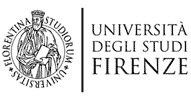Salta gli elementi di navigazioneDIMAI Dipartimento di Matematica e Informatica 'Ulisse Dini'
Menù principale

# Geometria

## Research topics

### Algebraic geometry

• Derived geometry
• DG-categories, Non-Commutative geometry, and applications to Arithmetic geometry, (Vanishing cycles, Singularity Theory, Bloch conductor conjecture)
• Geometric Langlands for surfaces
• K-theory for higher categories
• Representation theory of algebraic groups
• Fundamental groups
• Algebraic stacks
• Moduli spaces of vector bundles
• Group actions on varieties and on tensor spaces
• Geometry and identifiability of tensors
• Canonical decompositions of matrices with coefficients in a field, and applications
• Varieties of matrices: differential and algebraic properties
• Logarithms of real matrices
• Algebraic surfaces
• Syzygies of varieties
• LCI Algebraic Cycles
• Set theoretic complete intersection, Ideal theoretic complete intersection
• Geometric Complexity Theory
• Algebraic Statistics
• Computer formalization for Algebra and Geometry; Homotopy Type theory and Univalent Foundation

### Complex and hypercomplex analysis

• Geometric theory of holomorphic functions
• Spaces of holomorphic functions
• Monge-Ampère foliations and pluri-potential theory
• Holomorphic dynamics
• Automorphism groups and geometry of domains and complex manifolds, invariant metrics
• Hyperbolic geometry
• Modular forms
• Complex and hypercomplex analysis and geometry, with applications
• Slice-regular functions
• Quaternionic manifolds
• Orthogonal complex structures
• CAGD applications
• Computer formalization for Complex and Hypercomplex Analysis

### Complex, Symplectic, and Differential geometry

• Riemannian and pseudo-Riemannian geometry, Riemannian manifolds with prescribed sectional curvature
• Einstein metrics, Ricci solitons, Ricci flow with symmetries
• Homogeneous spaces, complex homogeneous spaces, and invariant structures on homogeneous spaces
• Norden and para-Norden geometry
• Complex and symplectic geometry, Generalized-complex geometries, Non-Kaehler geometry
• Complex Finsler Geometry
• Cohomology of complex manifolds, Hodge theory
• Special Hermitian metrics
• Riemannian geometry of space of Kaehler metrics
• Lagrangian foliations
• Convex geometry and toric Kaehler geometry
• Orbifold and quasifold structures, toric stratifications
• Holomorphic foliations, LVMB manifolds
• Complex structures in dimension 4
• Twistor methods
• Calibrated geometries
• Quaternionic manifolds
• Applications to Differential Geometry and to Computer Aided Geometric Design

### Combinatorics

• Weighted graphs

### Linear and multilinear algebra

• Matrix completion
• Decomposition of tensors, Real and complex ranks of tensors
• Spectral Theory of Tensors
• Identifiability of Tensors
• Canonical factorizations of matrices, Determinantal varieties, Secant varieties

### Foundations, didactic, popularization in Geometry

• Didactic aspects of Mathematics and popularization
• Mathematics, Music, and Architecture

## Postdocs

• Federico Caucci
• Francesco Pediconi
• Zahra Shahidi

## PhD students

• Giuseppe Barbaro
• Ettore Teixeira Turatti

## Other frequent collaborators

• Elena Angelini
• Alessandra Bernardi
• Cristiano Bocci
• Chiara Brambilla
• Luca Chiantini
• Juan Pablo Diaz Gonzalez
• Jan Draisma
• Benjamin Hennion
• JM Landsberg
• Valerio Melani
• Massimilano Mella
• Luke Oeding
• Francesco Panelli
• Mauro Porta
• Jasna Prezelj
• Alberto Raffero
• Marco Robalo
• Giulia Sarfatti
• Bernd Sturmfels
• Nicoletta Tardini
• Alicia Tocino
• Bertrand Toen
• Nick Vannieuwenhoven
• Alberto Verjovsky Sola
• Fabio Vlacci

## PhD Courses

ultimo aggiornamento: 12-Set-2020